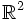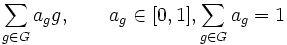# Tour:Interdisciplinary problems five (beginners)

UP: Introduction five |
PREVIOUS SECTION Interdisciplinary problems: Interdisciplinary problems four|NEXT SECTION Interdisciplinary problems: Interdisciplinary problems six
General instructions for the tour | Pedagogical notes for the tour | Pedagogical notes for this part

## Topology

Recall that a topological group is a group that is also a topological space, where the group operation and inverse map are both continuous.

### Actions of (discrete) groups on topological spaces

Suppose$G$ is a group (with no topology, or equivalently, with the discrete topology) and$X$ is a topological space. A topological group action of$G$ on$X$ is defined to be a group action in the set-theoretic sense, with the additional condition that for any$g \in G$, the permutation of$X$ induced by$g$ is a self-homeomorphism of$X$.

Equivalently, it is a homomorphism from$G$ to the group of self-homeomorphisms of$X$.

The action is termed faithful or effective if no non-identity element of$G$ acts as the identity on$X$.

Note that the actions here are only assumed to be topological, and need not preserve any of the further structure of the topological space.

1. Prove that the only cyclic groups of finite order that have a faithful action on the topological space$\R$ are the trivial group and the cyclic group of order two. (Hint: Use the ordering of the reals)
2. Prove that every cyclic group of finite order has a faithful action on the topological space$\R^2$. (Hint: Consider rotations).
3. Let$n$ be a natural number. Prove that the symmetric group of degree$n$ has a faithful group action on the topological space$X^n$ for any topological space$X$.
4. Prove that every finite group has a faithful group action on some Euclidean space (in the topological sense).
5. NEEDS LOT OF THOUGHT: Give an example of a finite group$G$ and a natural number$n$ such that$G$ has a faithful group action on$\R^n$ but$G$ does not have any faithful action on$\R^n$ that preserves the Euclidean distance.
6. Suppose$X$ is an infinite set endowed with the cofinite topology: the proper closed subsets are precisely the finite subsets. Prove that any group action of a group$G$ on$X$ is a topological group action.

### Actions of topological groups on topological spaces

Suppose$G$ is a topological group and$X$ is a topological space. A group action of$G$ on$X$ is termed a topological group action if the map$G \times X \to X$ associated with the action is continuous, where$G \times X$ has the product topology.

1. Prove that the action of a topological group on itself by left multiplication is a topological group action.
2. Suppose$\alpha:H \to G$ is a continuous homomorphism of topological groups, and$G$ has a topological group action on a topological space$X$. Construct a topological group action of$H$ on$X$.

## Measure theory

### A distribution over permutations

Suppose$G$ is the symmetric group of degree$n$, and$A$ is the set of all probability distributions over elements of$G$. A probability distribution over elements of$G$ is an expression of the form:$\sum_{g \in G} a_g g, \qquad a_g \in [0,1], \sum_{g \in G} a_g = 1$

1. Make$A$ a monoid by using the multiplication in the group, pulling out scalars, and collecting terms. (In particular, prove that the product defined this way also satisfies the conditions for being a probability distribution).
2. Determine the significance of this monoid from the viewpoint of probabilities.
3. Prove that in this monoid, the uniform distribution, defined as the distribution where all$a_g$ are equal to$1/n!$, is an idempotent element: it equals its own square.
4. NEEDS LOT OF THOUGHT: Find all idempotent probability distributions. (Hint: Relate these with subgroups)
5. Shuffle permutations: PLACEHOLDER FOR INFORMATION TO BE FILLED IN: [SHOW MORE]

## Category theory

The category of groups is the category whose objects are groups and such that the morphism set between two objects (groups) is the set of group homomorphisms between them. From the definition, it follows that isomorphisms in the category of groups are precisely the same as isomorphisms of groups.

### Categories with initial and terminal object

1. An initial object in a category is an object such that there is a unique morphism from it to any object. Prove that the trivial group is an initial object in the category of groups. (Since initial objects, if they exist, are unique up to isomorphism, the trivial group is the only initial object up to isomorphism in the category of groups).
2. A final object in a category is an object such that there is a unique morphism to it from any object. Prove that the trivial group is a final object in the category of groups.
3. A zero object in a category is an object that is both an initial and a final object. 'Prove that the trivial group is a zero object in the category of groups.
4. A trivial morphism or zero morphism in a category with a zero object is a morphism that is a composite of a morphism to the zero object and a morphism from the zero object. Prove that the trivial morphism between two groups is simply the homomorphism sending every element to the identity element.

### Products

1. Prove that the external direct product of two groups is their product in the categorical sense.
2. Construct a natural homomorphism from the coproduct of two groups in the categorical sense to their external direct product. (The coproduct in the categorical sense is called the free product of groups in group theory).